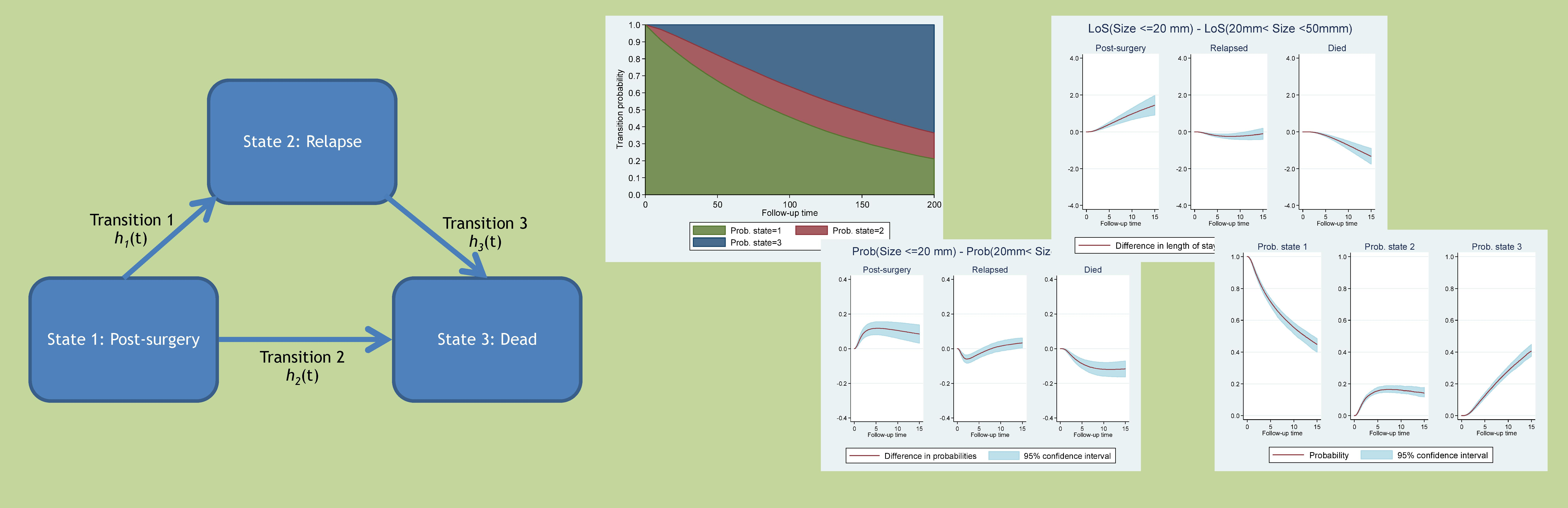Multistate

Multi-state models are increasingly being used to model complex disease profiles. By modelling transitions between disease states, accounting for competing events at each transition, we can gain a much richer understanding of patient trajectories and how risk factors impact over the entire disease pathway. In this project we concentrate on parametric multi-state models, both Markov and semi-Markov, and develop a flexible framework where each transition can be specified by a variety of parametric models including exponential, Weibull, Gompertz, Royston-Parmar proportional hazards models or log-logistic, log-normal, generalised gamma accelerated failure time models, possibly sharing parameters across transitions. We also extend the framework to allow time-dependent effects. We then use an efficient and generalisable simulation method to calculate transition probabilities from any fitted multi-state model, and show how it facilitates the simple calculation of clinically useful measures, such as expected length of stay in each state, and differences and ratios of proportion within each state as a function of time, for specific covariate patterns.

User-friendly Stata software has been developed, which can be downloaded within Stata by typing ssc install multistate.